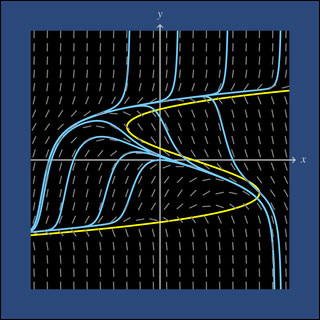18.03SC | Fall 2011 | Undergraduate

# Differential Equations

## Unit I: First Order Differential Equations

« Previous | Next »Integral curves and isoclines. Modeled on the MIT mathlet Isoclines.

In Unit I, we will study ordinary differential equations (ODE’s) involving only the first derivative.

y’ = F (x, y)

The first session covers some of the conventions and prerequisites for the course. After that we will focus on first order differential equations. We’ll start by defining differential equations and seeing a few well known ones from science and engineering. Very quickly we will learn about the three main ways of approaching ODE’s: Analytic (in symbols), geometric (with pictures and graphs), and numerical (with the computer).

A large part of this unit (and this course) will be spent on linear ODE’s. These are fundamental to the understanding of all ODE’s and among the most tractable.

« Previous | Next »

## Course Info

Fall 2011
##### Learning Resource Types
Lecture Videos
Recitation Videos
Exams with Solutions
Simulations
Lecture Notes
Problem Sets with Solutions